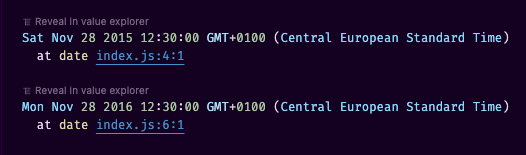# const - JS | lectureNumbers, Dates, Intl & Timers

Creating Dates

When we build real-world applications, one type of data that comes up all the time is dates and times. So let's learn the fundamentals of dates and times in this lecture so that we can put that into practice in the next one.

Dates and times can be a little bit messy and confusing in JavaScript. Hence, just like with numbers, I will try to make the fundamentals here as clear as possible.

Let's start by creating a date. There are exactly four ways of creating dates in JavaScript. They all use the constructor function, but they can accept different parameters. Let's see it in action.

The first way to create a date is by simply calling the `Date` constructor function without any parameters. This will create a date object that represents the current date and time.

script.js
``````const now = new Date();
console.log(now);``````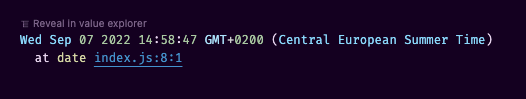The second way to parse a date from a date string.

script.js
``````// 👇🏽 Date string taken from the above console.log
console.log(new Date('Sep 07 2022 14:58:47')); // We will get the same date as above``````

When we provide a date string to JavaScript as we did above, it will then automatically parse the time based on that. We can, of course, also write a string ourselves.

script.js
``console.log(new Date('November 20, 1998'));``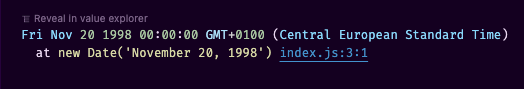You can see that it works, and we even get the day of the week. JavaScript is pretty smart when it comes to parsing date strings. However, it's generally not a good idea to parse dates from strings because it's not very reliable. But, if the string was actually created by JavaScript, then of course it is pretty safe.

Next, we can also pass in the year, month, day, hour minute and even second to the `Date` constructor function.

script.js
``````// You can also add the last parameter, the millisecond
console.log(new Date(1998, 10, 20, 12, 30, 45));``````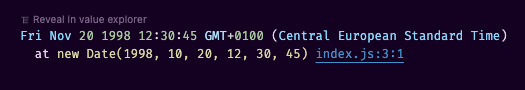You might have noticed that the second parameter which is the month is 10 but in the log in the console, it says November which is actually the 11th month. Hence, that means that the month parameter is zero-based. Yeah it's actually weird 😅, but we just have to get used to that.

Now what's cool about this is that JavaScript actually autocorrects the date for us. So if we pass in a date that doesn't exist, it will automatically correct it for us. For example, if we pass in the 31st of February, it will automatically correct it to the 3rd of March.

script.js
``console.log(new Date(2022, 1, 31));``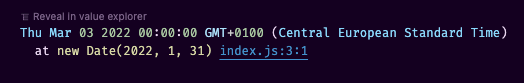Finally, we can also pass into the `Date` constructor function the number of milliseconds since January 1st, 1970. This is called the Unix Epoch.

script.js
``````/**
* Zero milliseconds since January 1st, 1970
*/
console.log(new Date(0));``````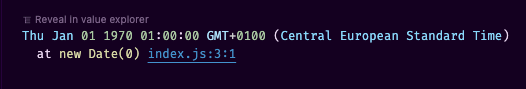Yeah, it feels a bit strange but with time and practice, you will get used to it. Now, let's create a date that is three days after the unix epoch.

script.js
``````/**
* 3 ==> 3 days
* 24 ==> A day has 24 hours
* 60 ==> An hour has 60 minutes
* 60 ==> A minute has 60 seconds
* 1000 ==> A second has 1000 milliseconds
*/
console.log(new Date(3 * 24 * 60 * 60 * 1000));``````The above number we calculated (`3 * 24 * 60 * 60 * 1000`) is called the timestamp or the timestamp of the day number 3. And we will see why this is useful later.

script.js
``console.log(3 * 24 * 60 * 60 * 1000); // 259200000``

Now, these dates that we just created are in fact, just another special type of object in JavaScript. Hence, they have their own methods, just like arrays, maps, or strings. And so we can use these methods to get or to set components of a date.

Let's start once more by creating a date and storing it in a variable.

script.js
``const date = new Date(2015, 10, 28, 12, 30);``

The first method we will look at is the `getFullYear` method. This method will return the year of the date.

script.js
``````console.log(date);
console.log(date.getFullYear()); // 2015``````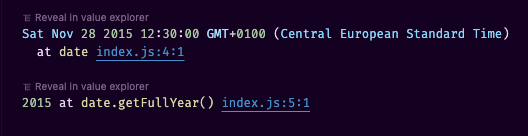There is also `getYear`, but never use that ‼️.

Next up, we have the `getMonth` method. This method will return the month of the date. However, it will return the month as a zero-based number.

script.js
``console.log(date.getMonth()); // 10``

Next, we have the `getDate` method.

script.js
``console.log(date.getDate()); // 28``

The method name is a bit misleading but that's actually the day of the month. To get the day of the week, we have the `getDay` method.

script.js
``console.log(date.getDay()); // 4``

Same as the month, the day of the week is also zero-based. Hence, 0 is Sunday, 1 is Monday, 2 is Tuesday, 3 is Wednesday, and so on.

Besides the day of the week, we can also get the hour, minute, second, and millisecond of a date.

script.js
``````console.log(date.getHours()); // 12
console.log(date.getMinutes()); // 30
console.log(date.getSeconds()); // 0
console.log(date.getMilliseconds()); // 0``````

We can also get a nicely formatted string similar to what we saw in the console when we logged the `date` variable.

script.js
``coonsole.log(date.toISOString()); // 2015-11-28T11:30:00.000Z``

Finally, we can also get the timestamp of a date. Remember that the timestamp is the number of milliseconds since January 1st, 1970.

script.js
``console.log(date.getTime()); // 1448710200000``

We can now get that timestamp and reverse it to get the date.

script.js
``console.log(new Date(1448710200000)); // This will give us the initial date we created above``

Timestamps are actually so important that there is a special method that we can use to get the current timestamp.

So if you want the current timestamp, you don't even need to create a new date. All you have to do is call the `Date.now` method.

script.js
``````/**
* This is my current timestamp so it will be different from yours
*/
console.log(Date.now()); // 1663154377916``````

To finish, there are also the `set` versions of the methods we saw above, and they also perform autocorrections just like when we create a new date. So for example, we can use the `setFullYear` method to set the year of a date.

script.js
``````date.setFullYear(2016);
console.log(date);``````